Hi there. My name is Noel and I work in Medical Sciences in Sydney Australia. I have been working with my Year 11 boy on probability and have now manufactured a problem which I cannot solve. The question relates to the following: I want to take all numbers in a Lotto game from 1 to 45 and ditribute them between 9 boxes each containing 5 numbers. Each number is used only once. What is the probability that I derive the correct combination of 5 numbers in the draw? What is the probability if I take a systems game and now distribute 9 numbers into each of 5 boxes? Once again, the numbers are used only once. Can you explain what formulae are used and how the answer is derived? Is this a distribution or combination problem? Or is it a combination of both distribution and combination? Thanx Noel Hi Noel, We will look at the 9 box version first. To start you have 45 numbers and you choose 5 of them to put in the first box. You can do this in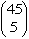ways. Once this is done you can choose 5 of the 40 remaining numbers to put in the second box in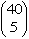ways. Thus the number of ways that you can choose 5 numbers for the first box and 5 numbers for the second box is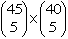Continuing in this fashion there are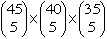ways to 5 numbers in each of the first 3 boxes and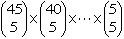ays to put 5 numbers in each of the 9 boxes. This number can be simplified to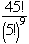which is approximately 2.32 x 1037 Once the 45 numbers have been placed in 9 boxes and the draw is about to take place, there are 9 draws that will result in you winning. Thus the probability that you win is approxinately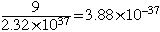Can you now see how to solve your second problem? Andrei and Penny Go to Math Central